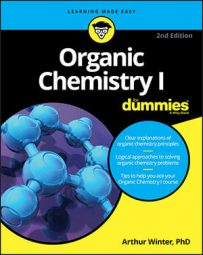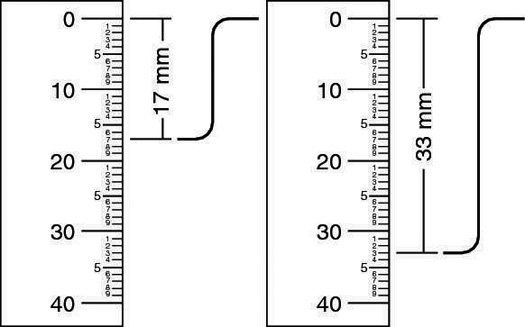##### Organic Chemistry I For DummiesHydrogen NMR (often called proton NMR or 1H NMR) is often the most useful NMR (nuclear magnetic resonance) type for organic chemists.

For example, the 1H NMR shows you the NMR peaks that represent particular functional groups in a molecular formula (specifically, carboxylic acids, aldehydes, and aromatic rings). You can then use integration ratios (plus information about the degrees of unsaturation and the molecule's location in the IR [infrared] spectrum) to determine all the fragments of the molecule.

## Compare integration ratios

You can determine how many hydrogens each NMR peak represents by comparing the integration ratios for a molecular compound to its molecular formula.

Using a ruler can be indispensable for finding out the relative integration ratios. What you need to measure with the ruler is the height of the integration curve over a given peak (the integration curve is the squiggle over a peak that looks like a capital S, as shown in the figure).

These heights are related to the areas underneath a peak, which in turn are related to the number of hydrogens that the particular peak represents. Determining how many hydrogens each peak represents will help you to find the fragments of the molecule later.Using a ruler to measure integration curves (for simplicity, the integration peaks aren't shown)
First, you want to establish the relative ratio between the integrations. To do this, use a ruler to measure the difference between the top and the bottom of each of the integration curves. After you measure the height of each peak on the spectrum, divide each of the heights by the smallest height. This will give you the ratios.

In this example, the smallest height is 17 mm, so you divide both heights by 17 mm. In this case, this gives an integration ratio of 1:1.94. Because you can never have a fraction of a hydrogen atom, you need to convert the relative ratios into whole numbers.

## Round to the nearest whole number

As is the case in this example, you often find that the integrations don't work out to be perfect whole numbers. If the numbers are very close to whole numbers, the integrations are probably just in error by a bit, and you simply need to round them to the nearest whole number. Because 1.94 is very close to 2.0, in this case you would say that the integration is 1:2.

For ratios that are not close to whole numbers, you need to multiply the ratio by the smallest number that makes both numbers in the ratios whole numbers. If the ratio were 1.0:1.5, for example, you would multiply both parts of the ratio by two to give a whole-number ratio of 2:3. If the ratio were 1.0:1.33, you would multiply both by three to give a whole-number ratio of 3:4, and so on.

So, for this example, you could say that your relative ratio of hydrogens is 1:2. Alternatively, using organic-speak, you could say that the peak on the left integrates for one and that the peak on the right integrates for two.